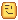# Check if ball is fully inside another ball

Hi
I want to make a function which checks if a ball is fully inside another ball. Ball sizes are different, I want to make it so that bigger balls can eat smaller balls like in Agar io so that the bigger ball has to cover the whole smaller ball if that makes sense.

According to the post shown below,

it uses inverse CFrame to detect if a part is inside each other.

``````function isInsideBrick(position, brick)
local v3 = brick.CFrame:PointToObjectSpace(position)
return (math.abs(v3.X) <= brick.Size.X / 2)
and (math.abs(v3.Y) <= brick.Size.Y / 2)
and (math.abs(v3.Z) <= brick.Size.Z / 2)
end
``````

Hope this helps1 Like

Thanks for replying, I tried it but it doesn’t seem to work too well with spheres.
Just to visualise it, I want to check whenever the red ball is inside the blue ball.

Try this. It checks if the ball is in the other ball using `Magnitude` and both of the balls’ sizes.

``````-- b1 is the base ball or the detector ball
-- b2 is the incoming ball that is being checked to see if it is all the way in b1
local function BallIsInOtherBall(b1, b2) -- (Ball, Ball)
if (b1.Position - b2.Position).Magnitude > b1.Size.Y/2 - b2.Size.Y/2 then
return false
else
return true
end
end
``````
1 Like

Thank you so much! It works perfectly!

1 Like